Courses

# Value Based Questions- Areas of Parallelograms and Triangles Class 9 Notes | EduRev

## Class 9 Mathematics by Full Circle

Created by: Full Circle

## Class 9 : Value Based Questions- Areas of Parallelograms and Triangles Class 9 Notes | EduRev

The document Value Based Questions- Areas of Parallelograms and Triangles Class 9 Notes | EduRev is a part of the Class 9 Course Class 9 Mathematics by Full Circle.
All you need of Class 9 at this link: Class 9

Question 1.A farmer was having a field in the form of a parallelogram PQRS. He divides it into three parts ∆PSA, ∆PAQ and ∆QAR such that AS = AR. He sows wheat in the triangular part PSA and donates the product to an orphanage.
(a) What part of the parallelogram PQRS is the triangular field PSA?
(b) Which mathematical concept is used in the above problem?
(c) By donating wheat product to an orphanage which value is depicted by the farmer?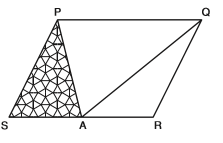Sol. (a) ∵ SA = AR [Given]
Also, SR ║ PQ                          [Opposite sides of ║gm PQRS]
∴ ∆PSA and ∆QAR are on the equal bases and between the same parallels SR and PQ.
⇒ ar (∆PSA) = ar (∆QAR)                       ...(1)
Since, ∆PAQ and ║gm PQRS are on the same base PQ and between the same parallels PQ and SR.
∵ ar (∆PAQ) = (1/2) ar (║gm PQRS)
⇒ ar (∆PSA) + ar (∆QAR) =(1/2) ar (║gm PQRS)                       ...(2)
From (1) and (2), we have
2 ar (∆PSA) =(1/2) ar (║gm PQRS)
⇒ ar (∆PSA) = (1/4)ar (║gmPQRS)
i.e., ∆PSA is one-fourth of ║gm PQRS.

(b) Areas of Parallelograms and Triangles

(c) Charity.

Question 2.A villager Itwari has a plot of land in the shape of a quadrilateral ABCD. The Gram Panchayat of the village plan to take over a portion of his plot from one of the corners to construct a charitable Health-Centre. Itwari agrees to the above proposal with a condition that he should be given equal amount of land in lieu of his land adjoining his plot so as to form a triangular plot.
(a) Explain how the above proposal will be implemented?
(b) Which mathematical concept has been covered in the above problem?
(c) Which values are depicted in the above plan?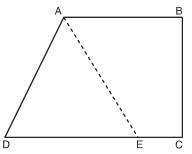Sol. (a) The given plot of Itwari is given by quadrilateral ABCD.
Let us draw DF ║ AC and join A and F.
Since, ∆DAF and ∆DCF are on the same base (DF) and between the same parallels (AC ║ DF)
∵ ar (∆DAF) = ar (∆DCF)
or ar (∆DAF) – ar (∆DEF) = ar (∆DCF) – ar (∆DEF)
⇒ ar (∆ADE) = ar (∆CEF)
Thus, the portion ∆ADE can be taken over by the Gram Panchayat by adding the land ∆CEF to this (Itwari’s) land so as to form it a triangular plot i.e., ∆ABF.

(b) Areas of Parallelograms and Triangles.

(c)(i) Community service (ii) Charity.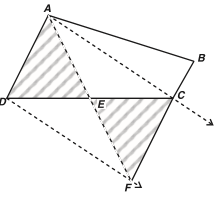Question 3.Ranjeet was having a field in the form of a parallelogram PQRS. He divides it into three parts by taking ‘A’ on RS and joining it to ‘P’ and ‘Q’. Such that : (i) One part is exactly half of the given field.

(ii) In the remaining two parts, he wants to sow wheat and pulses separately and donates their products to an orphanage.

(a) How the above plan can be implemented?

(b) Which mathematical concept is used in this problem?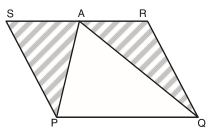(c) By donating the product of two parts wheat + pulses to an orphanage, which value is depicted by Ranjeet?
Sol.
(a) Here, Ranjeet is having the field in the form of ║gm PQRS. He marks a point ‘A’ on RS and joins AP and AQ.
Now, the field is divided into three parts i.e., ∆APS, ∆PAQ and ∆QAR.
∴ ∆PAQ and ║gm PQRS are on the same base (PQ) and between the same parallels (PQ ║ RS).
∵ ar (∆PAQ) = 1
2 ar (║gm PQRS) ...(1) Also, ar (∆APS) + ar (∆PAQ) + ar (∆QAR) = ar (║gm PQRS) ⇒ ar (∆APS) + 1
2 ar (║gm PQRS) + ar (∆QAR) = ar (║gm PQRS)
⇒ ar (∆APS) + ar (∆QAR) = 1
2 ar (║gm PQRS) ...(2)
⇒ From (1) and (2), we have ar (∆APS) + ar (∆QAR) = ar (∆PAQ)
Thus, the field is divided into three desired parts, i.e.,
(i) ∆APS,
(ii) ∆QAR and
(iii) ∆PAQ

(b) Areas of Parallelograms and Triangles. (c) Charity

Question 4.Rahul has a triangular plot ABC. He wants to divide it into 4 equal triangular parts. He donates one of the triangular part containing the vertex ‘A’ to residents welfare society for constructing a charity health centre unit.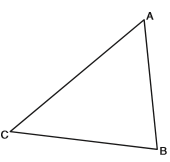(a) How the above plan can be implemented?
(b) What is the ratio of the donated part to remaining part of the plot?
(c) Do the remaining three parts taken together form a trapezium? If yes, then name it.
(d) Which mathematical concept is used in this problem?
(e) By donating a part of his plot for a charitable health centre, which value is depicted by Rahul?
Sol.
(a) Let us mark D, E and F as the mid-points of AB, BC and CA respectively. Since, in a ∆, line joining the mid points of any two sides is parallel to the third side and helf of it.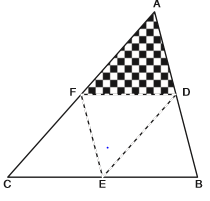⇒ EF║ AB and EF = (1/2)AB
∵ ∆ADF and ∆BDE are on equal bases (AB = BD) and between the same parallels (EF ║ AB) ⇒ ar (∆ADF) = ar (∆BDE)                       ...(1)
Similarly, ar (∆CEF) = ar (∆BDE)                       ...(2)
and ar (∆CEF) = ar (∆ADF)                      ...(3)
From (1), (2) and (3), we have ar (∆ADF) = ar (∆BDF) = ar (∆CEF)                       ...(4)
Also, ADEF is a ║gm and DF is a diagonal
∵ ar (∆ADF) = ar (∆DEF)                       ...(5)
From (4) and (5), we have ar (∆ADF) = ar (∆BDF) = ar (∆CEF) = ar (∆DEF)
Thus, the given triangular plot ABC is divided into 4 equal parts.
∵ The portion ∆ADF is donated to the welfare society.

(b) Since, out of 4 equal parts ∆ADF is 1 part.
∵ Remaining parts = 4 – 1 = 3
⇒ The required ratio is 1 : 3.

(c) ∴ DF ║ BC ∵ BCFD is a quadrilateral having one pair of opposite sides as parallel.
Thus, BCFD is a trapezium.

(d) Areas of Parallelograms and Triangles. (e) Charity and Social Service

Question 5.Praful has a piece of land ABCD which is in the form of a parallelogram. Ashok has a plot (triangular in shape) BOE adjoining to Praful’s land, such that AB = BE and BO = CO.
A welfare society plan to open an Adult-Education Centre in Ashok’s plot. Ashok agrees to exchange it with another triangular plot of same area. Praful allows to donate a triangular piece along DC to be exchanged with Ashok’s plot.
(a) How the above plan can be implemented?
(b) Which mathematical concept is involved in the above problem?
(c) By helping the welfare society in making its plan feasible, which values are depicted by Ashok and Praful?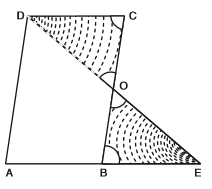Sol. (a) In the given figure ║gm ABCD is Praful’s piece of land and ∆BOE is Ashok’s plot.
Since, congurent triangles have same area.
∵ We have to cut off a ∆ along DC, which is congurent to ∆ BOE.
Join OD.
In ║gm ABCD, AB ║ DC and AB = DC                       [Opposite sides of a ║gm]
⇒ BE = DC                       [∴ AB = BE]              ...(1)
Now, in ∆BOE and ∆COD, we have ∠ OBE = ∠ OCD [Alternate interior angles]
∠ BOE = ∠ COD                       [Vertically opp. angles]
BE = DC                       [From (1)]
⇒ ∆BOE ≅∆COD                       [AAS]
⇒ ar (∆BOE) = ar (∆COD)
⇒ Thus, triangular pieces BOE and COD can be exchanged.

(b) Areas of Parallelograms and Triangles.

(c)(i) Charity (ii) Co-operation.

132 docs

,

,

,

,

,

,

,

,

,

,

,

,

,

,

,

,

,

,

,

,

,

;